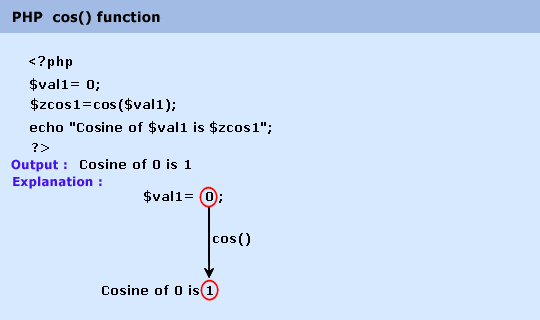# Education is not limited to just classrooms. It can be gained anytime, anywhere... - Ravi Ranjan (M.Tech-NIT)

## PHP : cos() function

Description

The cos() function is used to get the cosine of a number.

Version

(PHP 4 and above)

Syntax

cos(num)

Parameter

Name

Description

Required /
Optional

Type

num

A number.

Required

Float

Return value

The cosine of num.

Value Type : Float.

Pictorial PresentationExample :

1. "Cosine of \$val1 is \$zcos1

2.  ";echo "Cosine of \$val2 is \$zcos2
"

3. ;echo "Cosine of \$val3 is \$zcos3
";?>

Output :

Cosine of 0 is 1
Cosine of 4 is -0.653643620864
Cosine of -4 is -0.653643620864